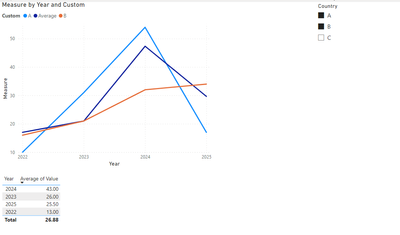cancel
Showing results for
Did you mean:Regular Visitor

## Problem with legend and measure in plot

Hello

I have a little problem with line chart and measure.

I have plot with measure (sum of values) on Y Y axis  and when I put on legend name of country I see couple of line depends which country I chosen on slicer , so it's right . But when I want put on plot another measure (Average of values for all country ) I couldnt do that .

so how solve that problem ?

I see solve the problem . I can make a lot of separate measure( calculate ...., Filter =Spain... etc )  for each country but it will be tough,

someone could help ?

1 ACCEPTED SOLUTIONCommunity Support

Based on your description, I have created a simple sample:First create a new table like this:

``````let
Source = Table.FromRows(Json.Document(Binary.Decompress(Binary.FromText("Vc6xDcAwCETRXahdGDBJ2iRjWOy/hk1ko0txzZO+oHeSKkKF7jmu5CXlCTlQ3jmxLboqZZSo5CdRcUpblTWUqFRQorK8bvvDE+WrGkpUepH7AA==", BinaryEncoding.Base64), Compression.Deflate)), let _t = ((type nullable text) meta [Serialized.Text = true]) in type table [Year = _t, Legend = _t, Value = _t]),
#"Changed Type" = Table.TransformColumnTypes(Source,{{"Year", Int64.Type}, {"Legend", type text}, {"Value", Int64.Type}}),
#"Removed Other Columns" = Table.SelectColumns(#"Changed Type",{"Legend"}),
#"Removed Duplicates" = Table.Distinct(#"Removed Other Columns"),
#"Expanded Custom" = Table.ExpandListColumn(#"Added Custom", "Custom"),
#"Renamed Columns" = Table.RenameColumns(#"Expanded Custom",{{"Legend", "Country"}})
in
#"Renamed Columns"``````Then build relationship between the two tables:Create a slicer:Apply the measure to the line chart:

``````Measure =
var _a = ALLSELECTED('Table'[Country])
return
IF(SELECTEDVALUE(Legend[Custom])="Average",CALCULATE(AVERAGE('Table'[Value]),FILTER(ALL('Table'),[Year]=SELECTEDVALUE('Table'[Year]))),SUM('Table'[Value]))``````

Final output:Best Regards,

Jianbo Li

If this post helps, then please consider Accept it as the solution to help the other members find it more quickly.Community Support

Based on your description, I have created a simple sample:First create a new table like this:

``````let
Source = Table.FromRows(Json.Document(Binary.Decompress(Binary.FromText("Vc6xDcAwCETRXahdGDBJ2iRjWOy/hk1ko0txzZO+oHeSKkKF7jmu5CXlCTlQ3jmxLboqZZSo5CdRcUpblTWUqFRQorK8bvvDE+WrGkpUepH7AA==", BinaryEncoding.Base64), Compression.Deflate)), let _t = ((type nullable text) meta [Serialized.Text = true]) in type table [Year = _t, Legend = _t, Value = _t]),
#"Changed Type" = Table.TransformColumnTypes(Source,{{"Year", Int64.Type}, {"Legend", type text}, {"Value", Int64.Type}}),
#"Removed Other Columns" = Table.SelectColumns(#"Changed Type",{"Legend"}),
#"Removed Duplicates" = Table.Distinct(#"Removed Other Columns"),
#"Expanded Custom" = Table.ExpandListColumn(#"Added Custom", "Custom"),
#"Renamed Columns" = Table.RenameColumns(#"Expanded Custom",{{"Legend", "Country"}})
in
#"Renamed Columns"``````Then build relationship between the two tables:Create a slicer:Apply the measure to the line chart:

``````Measure =
var _a = ALLSELECTED('Table'[Country])
return
IF(SELECTEDVALUE(Legend[Custom])="Average",CALCULATE(AVERAGE('Table'[Value]),FILTER(ALL('Table'),[Year]=SELECTEDVALUE('Table'[Year]))),SUM('Table'[Value]))``````

Final output:Best Regards,

Jianbo Li

If this post helps, then please consider Accept it as the solution to help the other members find it more quickly.